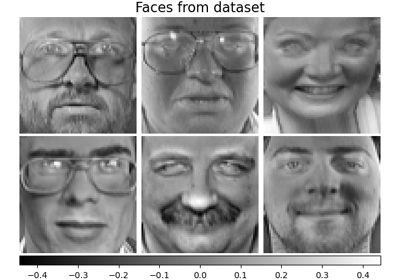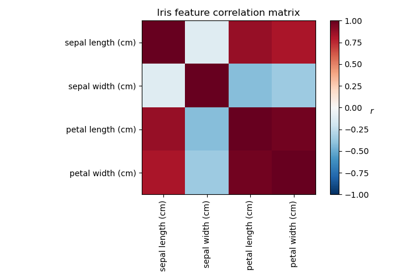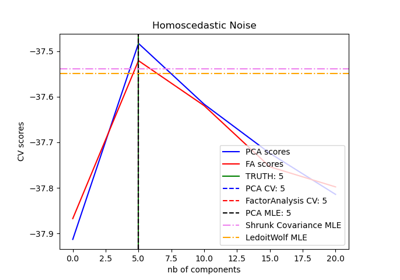# sklearn.decomposition.FactorAnalysis¶

class sklearn.decomposition.FactorAnalysis(n_components=None, *, tol=0.01, copy=True, max_iter=1000, noise_variance_init=None, svd_method='randomized', iterated_power=3, rotation=None, random_state=0)[source]

Factor Analysis (FA).

A simple linear generative model with Gaussian latent variables.

The observations are assumed to be caused by a linear transformation of lower dimensional latent factors and added Gaussian noise. Without loss of generality the factors are distributed according to a Gaussian with zero mean and unit covariance. The noise is also zero mean and has an arbitrary diagonal covariance matrix.

If we would restrict the model further, by assuming that the Gaussian noise is even isotropic (all diagonal entries are the same) we would obtain PCA.

FactorAnalysis performs a maximum likelihood estimate of the so-called loading matrix, the transformation of the latent variables to the observed ones, using SVD based approach.

Read more in the User Guide.

New in version 0.13.

Parameters:
n_componentsint, default=None

Dimensionality of latent space, the number of components of X that are obtained after transform. If None, n_components is set to the number of features.

tolfloat, default=1e-2

Stopping tolerance for log-likelihood increase.

copybool, default=True

Whether to make a copy of X. If False, the input X gets overwritten during fitting.

max_iterint, default=1000

Maximum number of iterations.

noise_variance_initndarray of shape (n_features,), default=None

The initial guess of the noise variance for each feature. If None, it defaults to np.ones(n_features).

svd_method{‘lapack’, ‘randomized’}, default=’randomized’

Which SVD method to use. If ‘lapack’ use standard SVD from scipy.linalg, if ‘randomized’ use fast randomized_svd function. Defaults to ‘randomized’. For most applications ‘randomized’ will be sufficiently precise while providing significant speed gains. Accuracy can also be improved by setting higher values for iterated_power. If this is not sufficient, for maximum precision you should choose ‘lapack’.

iterated_powerint, default=3

Number of iterations for the power method. 3 by default. Only used if svd_method equals ‘randomized’.

rotation{‘varimax’, ‘quartimax’}, default=None

If not None, apply the indicated rotation. Currently, varimax and quartimax are implemented. See “The varimax criterion for analytic rotation in factor analysis” H. F. Kaiser, 1958.

New in version 0.24.

random_stateint or RandomState instance, default=0

Only used when svd_method equals ‘randomized’. Pass an int for reproducible results across multiple function calls. See Glossary.

Attributes:
components_ndarray of shape (n_components, n_features)

Components with maximum variance.

loglike_list of shape (n_iterations,)

The log likelihood at each iteration.

noise_variance_ndarray of shape (n_features,)

The estimated noise variance for each feature.

n_iter_int

Number of iterations run.

mean_ndarray of shape (n_features,)

Per-feature empirical mean, estimated from the training set.

n_features_in_int

Number of features seen during fit.

New in version 0.24.

feature_names_in_ndarray of shape (n_features_in_,)

Names of features seen during fit. Defined only when X has feature names that are all strings.

New in version 1.0.

PCA

Principal component analysis is also a latent linear variable model which however assumes equal noise variance for each feature. This extra assumption makes probabilistic PCA faster as it can be computed in closed form.

FastICA

Independent component analysis, a latent variable model with non-Gaussian latent variables.

References

• David Barber, Bayesian Reasoning and Machine Learning, Algorithm 21.1.

• Christopher M. Bishop: Pattern Recognition and Machine Learning, Chapter 12.2.4.

Examples

>>> from sklearn.datasets import load_digits
>>> from sklearn.decomposition import FactorAnalysis
>>> transformer = FactorAnalysis(n_components=7, random_state=0)
>>> X_transformed = transformer.fit_transform(X)
>>> X_transformed.shape
(1797, 7)


Methods

 fit(X[, y]) Fit the FactorAnalysis model to X using SVD based approach. fit_transform(X[, y]) Fit to data, then transform it. Compute data covariance with the FactorAnalysis model. get_feature_names_out([input_features]) Get output feature names for transformation. get_params([deep]) Get parameters for this estimator. Compute data precision matrix with the FactorAnalysis model. score(X[, y]) Compute the average log-likelihood of the samples. Compute the log-likelihood of each sample. set_params(**params) Set the parameters of this estimator. Apply dimensionality reduction to X using the model.
fit(X, y=None)[source]

Fit the FactorAnalysis model to X using SVD based approach.

Parameters:
Xarray-like of shape (n_samples, n_features)

Training data.

yIgnored

Ignored parameter.

Returns:
selfobject

FactorAnalysis class instance.

fit_transform(X, y=None, **fit_params)[source]

Fit to data, then transform it.

Fits transformer to X and y with optional parameters fit_params and returns a transformed version of X.

Parameters:
Xarray-like of shape (n_samples, n_features)

Input samples.

yarray-like of shape (n_samples,) or (n_samples, n_outputs), default=None

Target values (None for unsupervised transformations).

**fit_paramsdict

Returns:
X_newndarray array of shape (n_samples, n_features_new)

Transformed array.

get_covariance()[source]

Compute data covariance with the FactorAnalysis model.

cov = components_.T * components_ + diag(noise_variance)

Returns:
covndarray of shape (n_features, n_features)

Estimated covariance of data.

get_feature_names_out(input_features=None)[source]

Get output feature names for transformation.

Parameters:
input_featuresarray-like of str or None, default=None

Only used to validate feature names with the names seen in fit.

Returns:
feature_names_outndarray of str objects

Transformed feature names.

get_params(deep=True)[source]

Get parameters for this estimator.

Parameters:
deepbool, default=True

If True, will return the parameters for this estimator and contained subobjects that are estimators.

Returns:
paramsdict

Parameter names mapped to their values.

get_precision()[source]

Compute data precision matrix with the FactorAnalysis model.

Returns:
precisionndarray of shape (n_features, n_features)

Estimated precision of data.

score(X, y=None)[source]

Compute the average log-likelihood of the samples.

Parameters:
Xndarray of shape (n_samples, n_features)

The data.

yIgnored

Ignored parameter.

Returns:
llfloat

Average log-likelihood of the samples under the current model.

score_samples(X)[source]

Compute the log-likelihood of each sample.

Parameters:
Xndarray of shape (n_samples, n_features)

The data.

Returns:
llndarray of shape (n_samples,)

Log-likelihood of each sample under the current model.

set_params(**params)[source]

Set the parameters of this estimator.

The method works on simple estimators as well as on nested objects (such as Pipeline). The latter have parameters of the form <component>__<parameter> so that it’s possible to update each component of a nested object.

Parameters:
**paramsdict

Estimator parameters.

Returns:
selfestimator instance

Estimator instance.

transform(X)[source]

Apply dimensionality reduction to X using the model.

Compute the expected mean of the latent variables. See Barber, 21.2.33 (or Bishop, 12.66).

Parameters:
Xarray-like of shape (n_samples, n_features)

Training data.

Returns:
X_newndarray of shape (n_samples, n_components)

The latent variables of X.

## Examples using sklearn.decomposition.FactorAnalysis¶Faces dataset decompositions

Faces dataset decompositionsFactor Analysis (with rotation) to visualize patterns

Factor Analysis (with rotation) to visualize patternsModel selection with Probabilistic PCA and Factor Analysis (FA)

Model selection with Probabilistic PCA and Factor Analysis (FA)